### Home > CAAC > Chapter 16 > Lesson 16.9.1.1 > Problem9-10

9-10.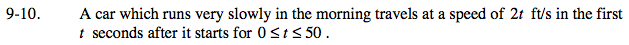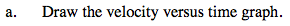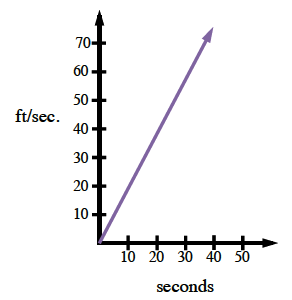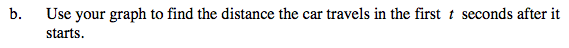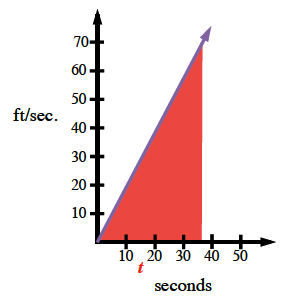$\text{Area}_\text{triangle}=\frac {\text{(base)(height)}}{2}$

Distance is the area under a velocity curve.

$\text{distance}=\frac{(t)(f(t))}{2}$

$\text{distance}=\frac{(t)(2t)}{2} = \frac{2t^{2}}{2} = t^2$